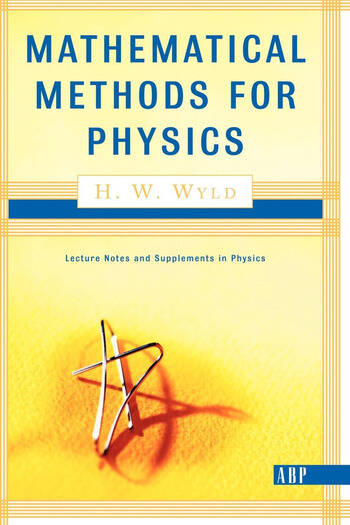# Mathematical Methods For Physics

## 1st Edition

H. W. Wyld, H.W. Wyld

CRC Press
Published March 31, 1999
Textbook - 652 Pages
ISBN 9780738201252 - CAT# K368175

For Instructors Request Inspection Copy

USD\$97.95

FREE Standard Shipping!

## Preview

### Summary

This classic book helps students learn the basics in physics by bridging the gap between mathematics and the basic fundamental laws of physics. With supplemental material such as graphs and equations, Mathematical Methods for Physics creates a strong, solid anchor of learning. The text has three parts: Part I focuses on the use of special functions in solving the homogeneous partial differential equations of physics, and emphasizes applications to topics such as electrostatics, wave guides, and resonant cavities, vibrations of membranes, heat flow, potential flow in fluids, plane and spherical waves. Part II deals with the solution of inhomogeneous differential equations with particular emphasis on problems in electromagnetism, Green's functions for Poisson's equation, the wave equation and the diffusion equation, and the solution of integral equations by iteration, eigenfunction expansion and the Fredholm series. Finally, Part II explores complex variable techniques, including evalution of itegrals, dispersion relations, special functions in the complex plane, one-sided Fourier transforms, and Laplace transforms.

#### Instructors

We provide complimentary e-inspection copies of primary textbooks to instructors considering our books for course adoption.